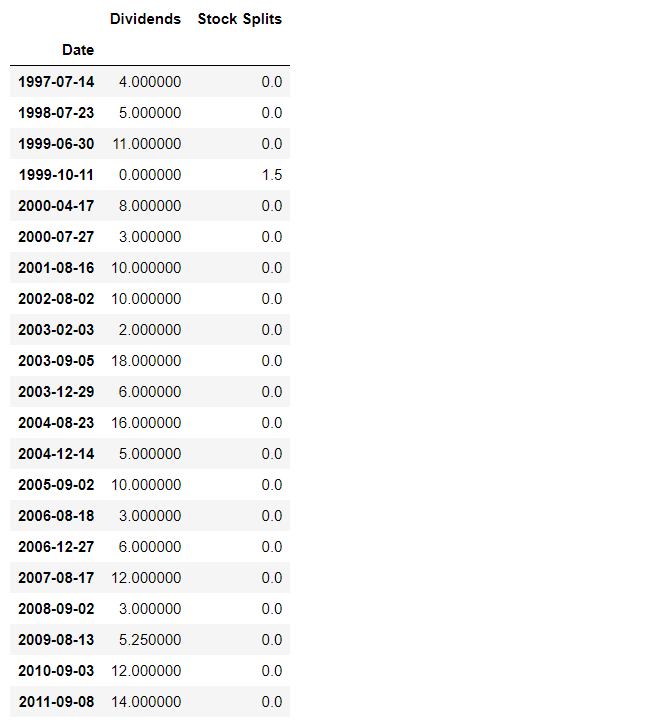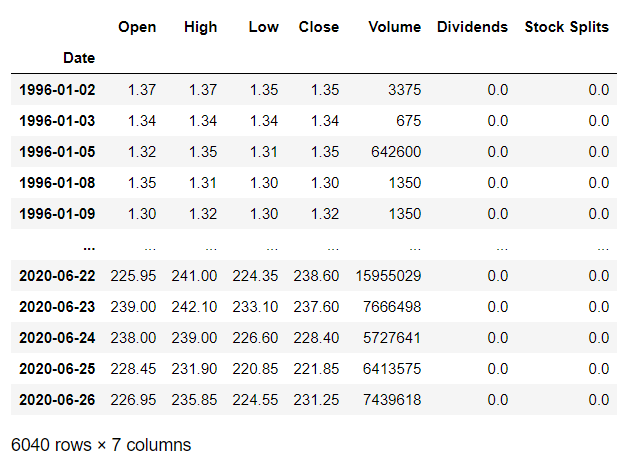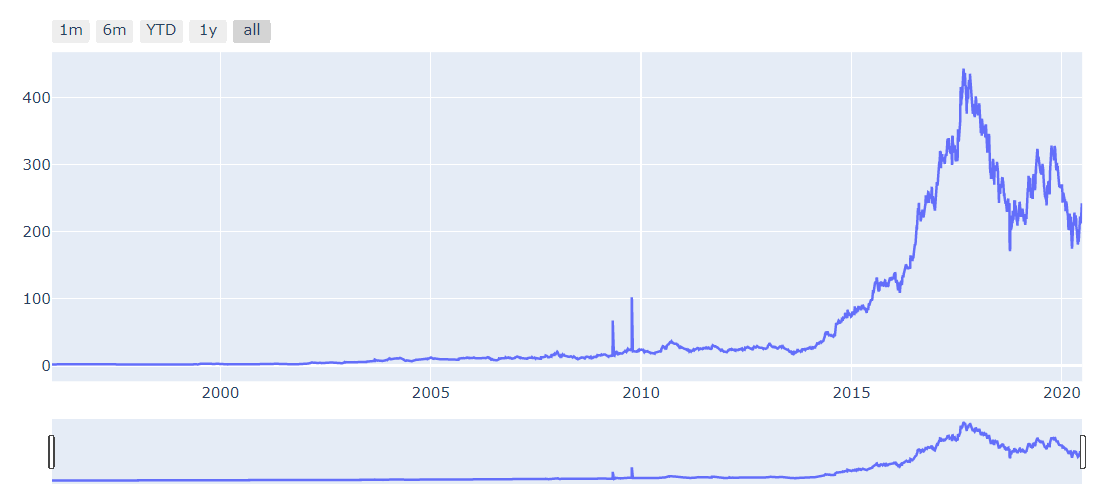Share :Originally posted on towardsdatascience.

Using YFinance and Plotly libraries for Stock Data Analysis

In the later part, we will see how we can use this data to plot different visually appealing and highly interactive financial charts using Plotly a python library. Plotly a Python library is an interactive, open-source plotting library that supports over 40 unique chart types covering a wide range of statistical, financial, geographic, scientific, and 3-dimensional use-cases.

Let’s Get Started. Initially, we will start by installing the YFinace library which we will use to download the stock data and see some of its features.

# Installing YFinance

Run the command given below in command prompt to install yfinance using pip.

`pip install yfinance`

# Exploring YFinance Library in Jupyter Notebook

Let’s start by importing the library and downloading the stock data. Here the stock ticker I am using is HINDPETRO.NS which is Hindustan Petroleum Corporation, you can choose any stock to analyze, just replace the stock ticker with your stock’s ticker.

`#importing Libraryimport yfinance as yf#setting the ticker hindpetro = yf.Ticker("HINDPETRO.NS")#Display stock informationhindpetro.info`

Now let us explore some functions that YFinance Library provides. This is just a small example there is much more, that you can explore here.

`# Dsiplay all the actions taken in the lifetime of the stock i.e    # dividends and splits with the dates when they are providedhindpetro.actions`Displaying the stock action i.e Dividends & Splits along with respective dates

Similarly, you can use the following given below commands to display the look at the stock dividends and stock split separately.

`#Display Dividendshindpetro.dividends#Display Splitshindpetro.splits`

Now let us download the data into a data frame and display it.

`df = hindpetro.history(period="max")df`Historical data of the HindPetro from the date it was listed until today.

For performing further operations we need to reset the index of the data frame and covert the respective columns to float datatype. Below given commands will solve our purpose.

`#Reseting the indexdf = df.reset_index()#Converting the datatype to floatfor i in ['Open', 'High', 'Close', 'Low']:    df[i] = df[i].astype('float64')`

After this, let us start with the visualization part. For this first, we will need to install Plotly.

# Installing Plotly

`pip install plotly`

# Creating a Line chart using Plotly Graph_objects with Range slider and button

A line chart is highly used for time series analysis, for viewing the stock trend over a time period. Here I will explain to you how you can create an interactive line chart using Plotly. The commands below will create a line chart of the stock data stored in the data frame over the maximum time period.

The code also includes the lines for creating buttons that can be selected to display a line chart for particular time periods.

`import plotly.graph_objects as goimport pandas as pdfig = go.Figure([go.Scatter(x=df['Date'], y=df['High'])])fig.update_xaxes(    rangeslider_visible=True,    rangeselector=dict(        buttons=list([            dict(count=1, label="1m", step="month",                                                         stepmode="backward"),            dict(count=6, label="6m", step="month",                   stepmode="backward"),            dict(count=1, label="YTD", step="year",                  stepmode="todate"),            dict(count=1, label="1y", step="year",                  stepmode="backward"),            dict(step="all")        ])    ))fig.show()`Line Chart for Stock Data with range selector and range selecting buttons.

# Creating OHLC(Open, High, Low, Close) Chart

An OHLC chart is a type of bar chart that shows open, high, low, and closing prices for each period. OHLC charts are useful since they show the four major data points over a period, with the closing price being considered the most important by many traders.

The code given below will create an OHLC chart with range selector.

# Creating a candlestick chart with the range slider

Candlestick charts are used by traders to determine possible price movements based on past patterns. Candlesticks are useful when trading as they show four price points (open, close, high, and low) throughout the period of time the trader specifies.

# Creating an area chart

An area chart or area graph displays graphically quantitative data. It is based on the line chart. The area between axis and line is commonly emphasized with colors, textures, and hatchings.

The code given below will create an area chart of the stock data.